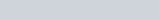Obtain the formula for the electric field due to a long thin wire of uniform linear charge density λ without using Gauss’s law.
Question:

Obtain the formula for the electric field due to a long thin wire of uniform linear charge density λ without using Gauss’s law. [Hint: Use Coulomb’s law directly and evaluate the necessary integral.]

Solution:

Take a long thin wire $X Y$ (as shown in the figure) of uniform linear charge density $\lambda$.Consider a point A at a perpendicular distance l from the mid-point O of the wire, as shown in the following figure.Let E be the electric field at point A due to the wire, XY.

Consider a small length element dx on the wire section with OZ = x

Let q be the charge on this piece.

$\therefore q=\lambda d x$

Electric field due to the piece,

$d E=\frac{1}{4 \pi \in_{0}} \frac{\lambda d x}{(\mathrm{AZ})^{2}}$

However, $A Z=\sqrt{\left(l^{2}+x^{2}\right)}$

$\therefore d E=\frac{\lambda d x}{4 \pi \in_{0}\left(l^{2}+x^{2}\right)}$

The electric field is resolved into two rectangular components. $d E \cos \theta$ is the perpendicular component and $d E \sin \theta$ is the parallel component.

When the whole wire is considered, the component $d E \sin \theta$ is cancelled.

Only the perpendicular component $d E \cos \theta$ affects point $\mathrm{A}$.

Hence, effective electric field at point $A$ due to the element $d x$ is $d E_{1}$.

$\therefore d E_{1}=\frac{\lambda d x \cos \theta}{4 \pi \epsilon_{0}\left(x^{2}+l^{2}\right)}$ …(1)

$\operatorname{In} \triangle \mathrm{AZO}$

$\tan \theta=\frac{x}{l}$

$x=l \tan \theta$   …(2)

On differentiating equation (2), we obtain

$d x=l \sec ^{2} \theta d \theta$

From equation (2),

$x^{2}+l^{2}=l^{2}+l^{2} \tan ^{2} \theta$

$\therefore l^{2}\left(1+\tan ^{2} \theta\right)=l^{2} \sec ^{2} \theta$

$x^{2}+l^{2}=l^{2} \sin ^{2} \theta$  …(4)

Putting equations (3) and (4) in equation (1), we obtain

$\therefore d E_{1}=\frac{\lambda l \sec ^{2} d \theta}{4 \pi \epsilon_{0} l^{2} \sec ^{2} \theta} \times \cos \theta$

$\therefore d E_{1}=\frac{\lambda \cos \theta d \theta}{4 \pi \in_{0} l}$ …(5)

The wire is so long that $\theta$ tends from $-\frac{\pi}{2}$ to $+\frac{\pi}{2}$.

By integrating equation (5), we obtain the value of field E1 as,

$\int_{-\frac{\pi}{2}}^{\frac{\pi}{2}} d E_{1}=\int_{-\frac{\pi}{2}}^{\frac{\pi}{2}} \frac{\lambda}{4 \pi \in_{0} l} \cos \theta d \theta$

$E_{1}=\frac{\lambda}{4 \pi \in_{0} l}[\sin \theta]_{-\frac{\pi}{2}}^{\frac{\pi}{2}}$

$=\frac{\lambda}{4 \pi \in_{0} l} \times 2$

$E_{1}=\frac{\lambda}{2 \pi \in_{0} l}$

Therefore, the electric field due to long wire is $\frac{\lambda}{2 \pi \epsilon_{0} l}$.